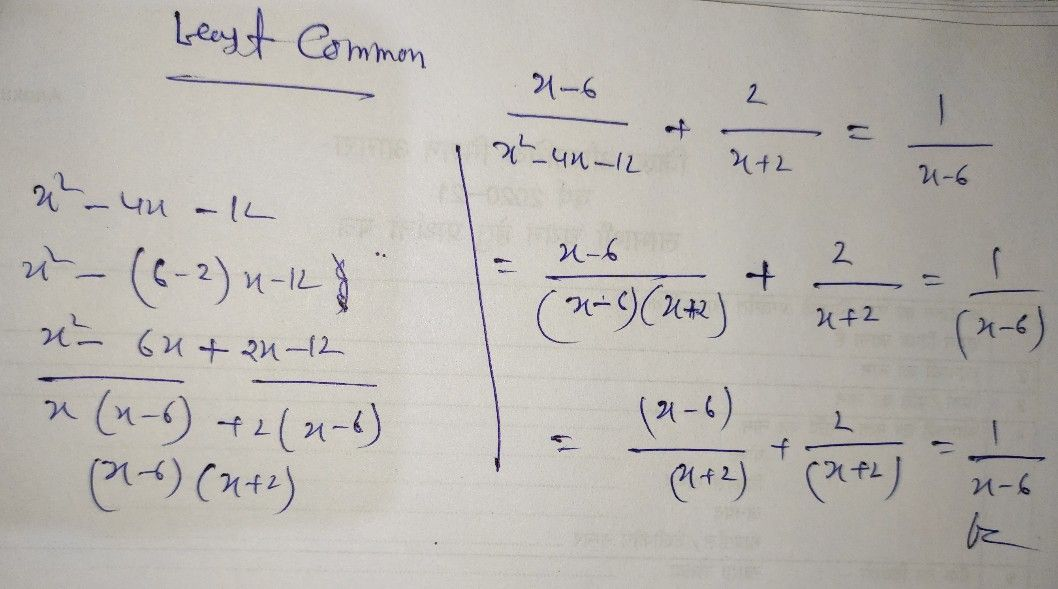Symbol
Problemmue By substituting $ny$ to the original equation and reject extrancous $oo1/=$ $2$ Rational Equation $\dfrac {x-6} {x^{2-4x-12}}+\dfrac {2} {x+2}=\dfrac {1} {x-6}$ 1. Find the Least Common Denominator (LCD). 2. Multiply both sides of the equation by $ts$ the LCD. 3. Apply the Distributive Property and then simplify. 4. Find all the possible values of $X$ $x=10$ $5$ Check each value $by$ substituting into original equation and reject $any$ extraneous $root/s$